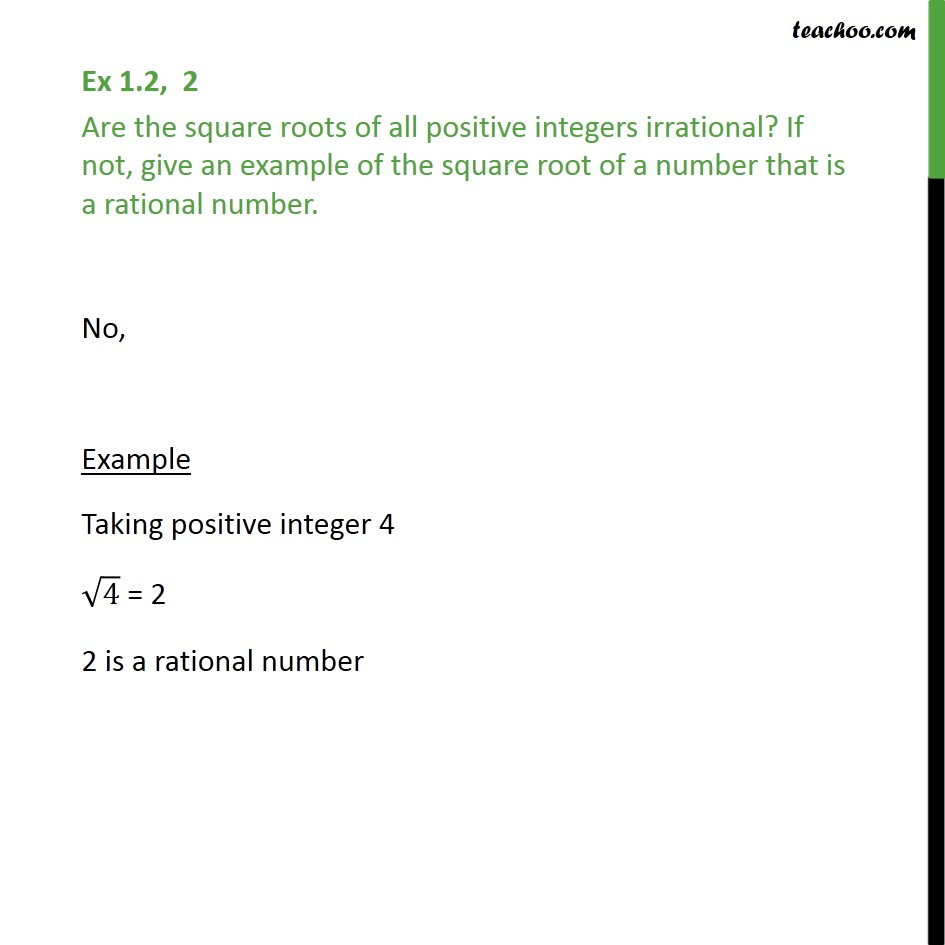Ex 1.2

Chapter 1 Class 9 Number Systems
Serial order wiseGet live Maths 1-on-1 Classs - Class 6 to 12

### Transcript

Ex 1.2, 2 Are the square roots of all positive integers irrational? If not, give an example of the square root of a number that is a rational number. No, Example Taking positive integer 4 4 = 2 2 is a rational number